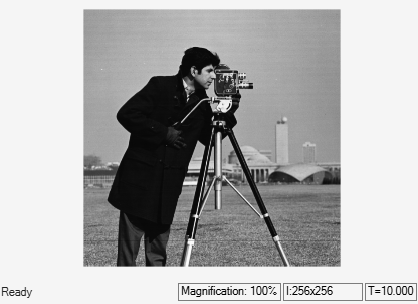# Median based Image Thresholding

This example shows how to perfom image thresholding with the median value of the image as a global threshold.

### Example Model

```open_system('ex_blk2dmedian.slx'); ```The model computes the median value of an input image by setting the `Find the median value over` parameter to `Entire` `input` in `2-D` `Median` block. The output of the `2-D` `Median` block is a scalar. The `Matlab` `Function` block performs image thresholding by taking the output median as a global threshold. If the intensity value in the input image is greater than the median value, it is set to '1'. Otherwise, the intensity value is set to '0'. The output of the model is the thresholded image.

### Simulate and Display Results

Run the model and display the results using `Video` `Viewer` block.

```sim('ex_blk2dmedian.slx'); ```